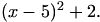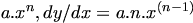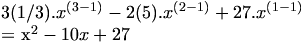Warning: Illegal string offset 'html' in /home/hsn/public_html/forum/cache/skin_cache/cacheid_1/skin_topic.php on line 909

Maths Q - HSN forum# Maths Q

20 replies to this topic

### #1Rocky

• Gender:Male

Posted 26 October 2005 - 03:58 PM

Q- The point A has co-ords (7,4). The straight line with equations x+3y+1=0 & 2x+5y=0 and they intersect at B.
(a) Find the gradient of AB
(b) Hence show that AB is perpendicular to only one of these 2 lines.

*Help will be appreciated, thanks.

### #2Rocky

• Gender:Male

Posted 26 October 2005 - 04:37 PM

Hello? Like I'm not saying you need to work it out to give me solutions. Just tell me what I need to do, to get the right answers. So please help me.

### #3dondon

HSN Legend

•• Members
•• 2,888 posts
• Location:Glasgow
• Gender:Female

Posted 26 October 2005 - 04:42 PM

a) use simultaneous equations, then work out the gradient from the coordinates.

b)then work out the gradients of both the lines and then see if they are perpendicular.

eg. if the gradient of AB was 3, the gradient of the perpindicular line would be -1/3

### #4Rocky

• Gender:Male

Posted 26 October 2005 - 04:57 PM

After I do Simul.Eqns with the 2 functions. I will then get the X&Y values, but what do I do with them to work out the gradients with the co-ords (7,4)??

### #5Steve

Top of the Class

••• 435 posts
• Location:Edinburgh
• Gender:Male

Posted 26 October 2005 - 04:59 PM

What you will have worked out is the coordinates of B, which you can use with the coordinates of A to find the gradient of the line AB.
HSN contribute: Help the site grow!

Looking for a Maths tutor in West Lothian? Just PM me!

### #6dondon

HSN Legend

•• Members
•• 2,888 posts
• Location:Glasgow
• Gender:Female

Posted 26 October 2005 - 04:59 PM

the x and y values are the x and y coordinates. so then you just use them and (7,4) in the gradient equation like you normally would.

### #7Rocky

• Gender:Male

Posted 26 October 2005 - 05:08 PM

So B is (8, -3) what do I do now? Do I do:
y2-y1 OVER x2-x1? But for the B co-ords or Aco-ords?

### #8dondon

HSN Legend

•• Members
•• 2,888 posts
• Location:Glasgow
• Gender:Female

Posted 26 October 2005 - 05:11 PM

yup you use that equation but with both the coordinates because they are 2 points on the line, so you can work out the gradient with them.

### #9Rocky

• Gender:Male

Posted 26 October 2005 - 05:16 PM

Oh silly me, need to open my eyes lol
Ok I get M=7, so now what do I do with the 7 for part (b). As you saying I need to see what line (out of the 2) will be perpendicular, so do I sub X=-7 into the 2 functions?

### #10dondon

HSN Legend

•• Members
•• 2,888 posts
• Location:Glasgow
• Gender:Female

Posted 26 October 2005 - 05:19 PM

nope you work out the gradients for the 2 equations, and they are -2 and -1, and you work out which line is perpindicular to AB using gradients.

### #11Rocky

• Gender:Male

Posted 26 October 2005 - 05:23 PM

So what other points do I use along with the -2 and -1?

### #12dondon

HSN Legend

•• Members
•• 2,888 posts
• Location:Glasgow
• Gender:Female

Posted 26 October 2005 - 05:29 PM

do you not know how to work out if lines are perpindicular??

you take the the gradient of each line say the first one was -2, for AB to be perpindicular it would need to be 1/2.

Because you turn the gradient round.

### #13Rocky

• Gender:Male

Posted 26 October 2005 - 05:33 PM

So if M= -7, then perpendicular line should be 1/7. But those lines don't have that, as you just shown so what do I do then?

### #14dondon

HSN Legend

•• Members
•• 2,888 posts
• Location:Glasgow
• Gender:Female

Posted 26 October 2005 - 05:42 PM

Actually the gradients are: -2/5 and -1/3, does that help at all??
If not i think maybe youve maybe copied it down wrong.

### #15Rocky

• Gender:Male

Posted 26 October 2005 - 05:44 PM

Ok, forget it. I'll somehow solve this Q, thanks for the help.

### #16dfx

Fully Fledged Genius

•• Members
•• 1,955 posts
• Gender:Male

Posted 26 October 2005 - 06:18 PM

(a) Let's work out point B:

x + 3y + 1 = 0 ... (i)
2x + 5y = 0 ... (ii)

From (i)
x = -1 - 3y
Then
2(-1 - 3y) + 5y = 0
-2 - y = 0 [=>0 y = -2

From (ii)
2x + 5(-2) = 02x = 10 then x = 5

so B is (5,-2)

M AB (the gradient of AB) is calculated to be 3

(b) Let's get the gradients of the two lines.

Let L1 be x+3y+1=03y = -x - 1m = -1/3 . Let's call this m1.

Let L2 be 2x+5y=05y = -2xm = -2/5 . Let's call this m2.

One of these lines (L1 or L2) is perpendicular to AB. Only way to find out is to compare the gradient of AB ( m ab) with those of L1 and L2 (m1 and m2), using the formula:

mPmQ = -1 (for perpendicular lines) ... we commonly know this as "flip the gradient and change the sign for perpendicular lines." (where mP and mQ are the two gradients)

Anyway, test using that and you see that only L1 is perpendicular to AB, and not L2.

### #17Rocky

• Gender:Male

Posted 27 October 2005 - 03:14 PM

Thanks but I got the answer just like you did. But can someone help as I am very frustrated with a little part in a Q, I need to do:

The Q was that write x2-10x+27 in the form (x+b)2 +c.
Which is completing the square, and I got (x-5)2 +2.
So all good, not exactly, as it then PART B says
"Hence show that g(x)=1/3(x-cubed) -5(x-squared)+27x-2 is always increasing"
Well I differentiated it kk and when I am trying to get the x values, it does not factorise!! So anyone can help me on this problem please.

### #18dfx

Fully Fledged Genius

•• Members
•• 1,955 posts
• Gender:Male

Posted 27 October 2005 - 03:19 PM

That's the whole point of getting you to complete the square, don't you see. Once you differentiate g(x) you end up with the previous function they asked you to complete the square with. Now you can rewrite the derivative the formFrom that you can get the turning points - look it up in your textbook.

### #19Rocky

• Gender:Male

Posted 27 October 2005 - 03:36 PM

But look when I differentiate it:
g(x)= 1/3(x-cubed) -5(x-squared)+27x-2

g-1(x)= 2/3(x-squared) -10x+27 (NOW I MULTIPLY BY 3)
2(x- squared) -30x+81=0

*Yeah it is very similar to my "completing the square" Eqn.
Plus it doesn't even bloody factorise properly.

### #20dfx

Fully Fledged Genius

•• Members
•• 1,955 posts
• Gender:Male

Posted 27 October 2005 - 04:29 PM

g-1(x)= 2/3(x-squared) -10x+27

You've differentiated wrong.Remember for:Differentiating:#### 1 user(s) are reading this topic

0 members, 1 guests, 0 anonymous users